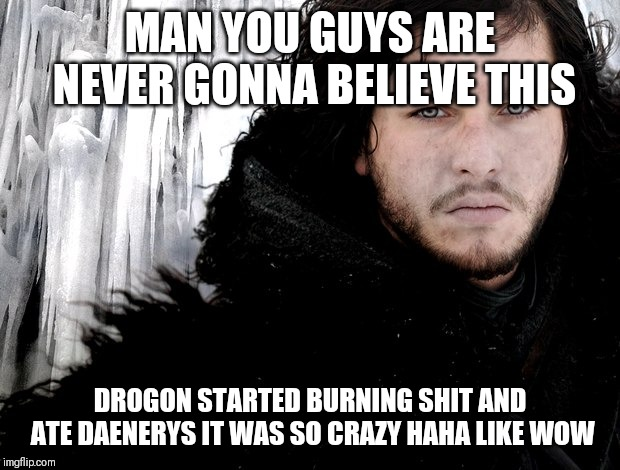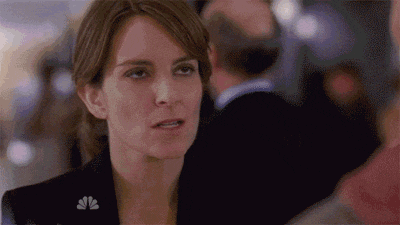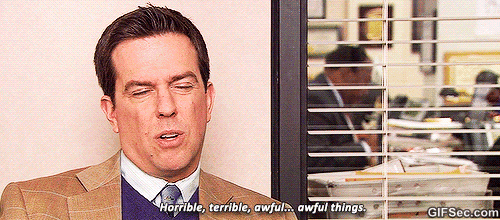# Thread: Game of Thrones Speculation

1. ##Reply With Quote

2. ##................... Is what I would have said

Bleepepepgpdjejujfhjdueiitjsiijshrji gotjjdjjrhhhxhduwiis djjr. Fhr ktjnospoilershere

Episode went from super dark to super light really, really fast. But, as always, fuck you Bran. I did lol at the 'let's try democracy' bit though.
Last edited by Taernath; 05-20-2019 at 02:32 AM.Reply With Quote

3. ##ffffffffffffffffffffffffffffffffffffffffffffffffff ffffffffffffffffffffffffffffffffffffffffffffffffff ffffffffffffffffffffffffffffffffffffffffffffffffff ffffffffffffffffffffff

Overall I'd say it was somewhat disappointing. I was happy that Ghost and Jon got reunited (and Ghost finally got pets, WTF Jon), Sansa got what she wanted, and Jon didn't actually get stuck back on the night's watch. The ending scene with Tyrion just seemed really out of place. Also, during the let's name a king part, I yelled out loud "Get fucked Edmure" when Sansa told him to sit down, so there's that.

Could have been worse, but it sure as fuck could have been a lot better. Also, Bran is a cold motherfucker, letting all that happen just to become king.Reply With Quote

4. ##Reply With Quote

5. ##They very clearly blew the CGI budget in the first thirty minutes and the rest of it was basically a high school play in terms of writing and acting just lmao. Garbage from the dump.Reply With Quote

6. ##Originally Posted by audioserfThey very clearly blew the CGI budget in the first thirty minutes and the rest of it was basically a high school play in terms of writing and acting just lmao. Garbage from the dump.
ffffffffffffffff ffffffffffffffffffffff fffffffffffffffffffffffffff fffffffffffffffffffffffffffffffff f f f ffffffffffffffffffffffffffff f fffffffffffffffffffffffffffffff

Nah it was well acted, just a much happier ending than the setting deserved.Originally Posted by VelfiAlso, during the let's name a king part, I yelled out loud "Get fucked Edmure" when Sansa told him to sit down, so there's that.
I didn't recognize anyone except for the main characters and that one fat guy in the council scene. Edmure is totally the fantasy Jeb! of the GoT universe.Reply With Quote

7. ##Last edited by Parkbandit; 05-20-2019 at 11:42 AM.Reply With Quote

8. ##Did they undo the Thanos snap?Reply With Quote

9. ##ffffffffffffffff ffffffffffffffffffffff fffffffffffffffffffffffffff fffffffffffffffffffffffffffffffff f f f ffffffffffffffffffffffffffff f fffffffffffffffffffffffffffffff

ffffffffffffffff ffffffffffffffffffffff fffffffffffffffffffffffffff fffffffffffffffffffffffffffffffff f f f ffffffffffffffffffffffffffff f fffffffffffffffffffffffffffffff

ffffffffffffffff ffffffffffffffffffffff fffffffffffffffffffffffffff fffffffffffffffffffffffffffffffff f f f ffffffffffffffffffffffffffff f fffffffffffffffffffffffffffffff

I knew it was going to happen, Jon killing Dany, but I was still disappointed with how it played out.

I enjoyed the dragon torching the iron throne.

I didn't expect Bran to become King.

Overall, it feels like a failure considering everything that took place up to the final season.
Last edited by Alastir; 05-20-2019 at 12:27 PM.Reply With Quote

10. ##Originally Posted by Taernathffffffffffffffff ffffffffffffffffffffff fffffffffffffffffffffffffff fffffffffffffffffffffffffffffffff f f f ffffffffffffffffffffffffffff f fffffffffffffffffffffffffffffffReply With Quote
bob the stalker, sssssssuckin squid dick, tisket sharted again